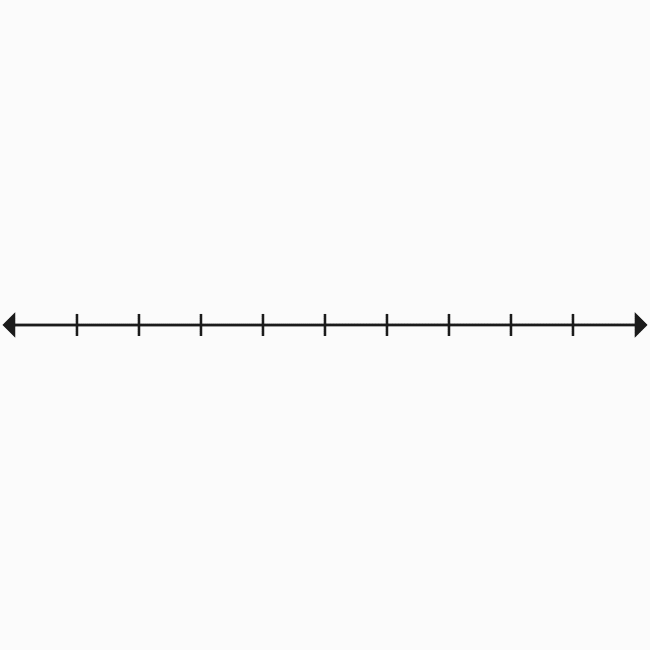# Origin of Two dimensional space

A point where the perpendicular number lines get bisected is called the origin of two dimensional space.

## Introduction

The two dimensional Cartesian coordinate system is actually formed by the perpendicular bisection of two number lines (or coordinate axes).The coordinate axes are bisected perpendicularly at their middle point and their bisecting point is considered as a starting point for determining the position of any point on the plane. Hence, the perpendicular bisecting point of the coordinate axes is called the origin of two dimensional space.

### Example

In this example, the coordinate axes are $x$-axis and $y$-axis. The two coordinate axes are perpendicularly bisected at point $O$.

The point $O$ is called the origin of bi-dimensional Cartesian coordinate system and it is used as a starting point for determining the position of any point in the plane.

Latest Math Topics
Jun 26, 2023
Jun 23, 2023

Latest Math Problems
Jul 01, 2023
Jun 25, 2023
###### Math Questions

The math problems with solutions to learn how to solve a problem.

Learn solutions

Practice now

###### Math Videos

The math videos tutorials with visual graphics to learn every concept.

Watch now

###### Subscribe us

Get the latest math updates from the Math Doubts by subscribing us.